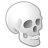# Arcade scripting in Attribute Rules

645
19
04-01-2021 11:15 AMOccasional Contributor

Hi All,

I've gotten over my hurtle with creating attribute rules now, but I'm a newbie with Arcade - either in the WAB or in Pro.

I'm attempting to create a Calculation rule that is based on If Then Else statements.  I am getting a message about Invalid expression.  Error on line 8.  Open parenthesis expected.

Here's the beginning of my expression; at the end I do have return eru;  As this is the field that I am attempting to calculate.  There will be three of these set up so as to do the required pre-calcs before getting the last two calculations that are the real ones to be returned and saved.

It is highly likely that I'm missing just something simple, I thought that I was following the examples that I found for doing calcs and using if statements.  But ....

var fs = FeatureSetByName(\$datastore,"IDF_Stormwater");
var billclass = fs["MultiUserType"];
var eru = fs["ERUArea"];
var parea = fs["IDFArea"];
var pimparea = fs["ImperviousArea"];
var dwell = fs["Dwellings"];

if billclass == 'D'
eru = 0
else if billclass in ('V','I','H')
eru = Round(parea/43560,2)
else if billclass == 'R'
eru = Round(parea*(2000/7760)/2000,2)
else if billclass in ('A','O')

.....

Thanks,

Lorinda

Tags (3)
19 RepliesbyMVP Regular Contributor

You're missing a statement after the last.else if.Occasional Contributor

Not really, the remainder of the script is after a few more lines:

else if billclass = 'R' && pimparea = 0
eru = Round(parea*(2000/7760)/2000,2)
else if billclass = 'R' && pimarea > 0
eru = (Round(pimparea/2000,2)

return eru;byMVP Esteemed Contributor

``````else if billclass = 'R' && pimparea = 0  /* Could this be a problem */
eru = Round(parea*(2000/7760)/2000,2)
else if billclass = 'R' && pimarea > 0   /* as well as this */
eru = (Round(pimparea/2000,2)

return eru;

/*
else if billclass == ('R' && pimparea = 0)
...
else if billclass == ('R' && pimarea > 0)
*/``````

can't wait to retire....byMVP Regular Contributor

@LorindaGilbert wrote:

Not really, the remainder of the script is after a few more lines:

Which is where the error is.byMVP Esteemed Contributor
``````var fs = FeatureSetByName(\$datastore,"IDF_Stormwater");
var billclass = fs["MultiUserType"];
var eru = fs["ERUArea"];
var parea = fs["IDFArea"];
var pimparea = fs["ImperviousArea"];
var dwell = fs["Dwellings"];

if billclass == 'D'
eru = 0
else if billclass in ('V','I','H')
eru = Round(parea/43560,2)
else if billclass == 'R'
eru = Round(parea*(2000/7760)/2000,2)
else if billclass in ('A','O')
//// Need something here as Luke points out``````

I took the liberty to copy and paste your code into the code editor; makes it easier to read.  If you expand the three dots to expand the tool bar and then choose the  "Insert/Edit  Code Sample" tool that looks like this: </> you can format your code as it is meant to be read.  I used JavaScript for this...

can't wait to retire....Occasional Contributor

My full script is this:

var fs = FeatureSetByName(\$datastore,"IDF_Stormwater");
var billclass = fs["MultiUserType"];
var eru = fs["ERUArea"];
var parea = fs["IDFArea"];
var pimparea = fs["ImperviousArea"];
var dwell = fs["Dwellings"];

if billclass == 'D'
eru = 0
else if billclass in ('V','I','H')
eru = Round(parea/43560,2)
else if billclass == 'R'
eru = Round(parea*(2000/7760)/2000,2)
else if billclass in ('A','O')
eru = Round(pimparea/2000,2)
else if billclass == 'M' && dwell in (1,2)
imparea = parea*0.3192
else if bill class == 'M' && dwell in (3,4)
imparea = parea*0.5093
else if billclass == 'M' && dwell > 4
imparea = parea*0.5613
else if billclass in ('M','A','O') && pimparea = 0
eru = Round(pimpara/2000,2)
else if billclass = 'R' && pimparea = 0
eru = Round(parea*(2000/7760)/2000,2)
else if billclass = 'R' && pimarea > 0
eru = (Round(pimparea/2000,2)

return eru;

Yes, I see that I am missing an end if it is needed.byMVP Regular Contributor

eru = (Round(pimparea/2000,2)

return eru;

Either remove the bracket before Round or add a closing bracket.byMVP Esteemed Contributor

Good Catch @Luke_Pinner !

can't wait to retire....Occasional Contributor

Alas, that didn't solve the expected open parenthesis error.

Haven't checked what Joe Borgione mentioned with the two items on one line yet and the &&.  Had to start on another project in FME right now.  Will try this again tomorrow.